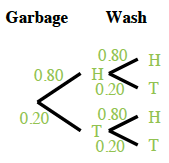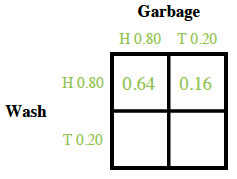### Home > GC > Chapter 5 > Lesson 5.1.1 > Problem5-9

5-9.

Earl hates to take out the garbage and to wash the dishes, so he decided to make a deal with his parents: He will flip a coin once for each chore and will perform the chore if the coin lands on heads. What he doesn’t know is that his parents are going to use a weighted coin that lands on heads $80$% of the time! Homework Help ✎

1. What is the probability that Earl will have to do both chores?What is the probability of flipping two heads in a row?

$(0.80)(0.80)=0.64=64\%$

2. What is the probability that Earl will have to take out the garbage, but will not need to wash the dishes?

Use a tree diagram or two-way table.

$(0.80)(0.20)=0.16=16\%$